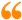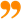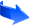﻿ The Duality of Time Theory#### 4.2 The Two Arrows of Time:

Discreteness implies interruption or discontinuity, and this is what the outer time is doing to the continuous flow of the inner time that is perpetually re-creating space and matter in one chronological sequence. Mathematically, this is achieved by multiplying with the imaginary unit, which produces an “abrupt rotation” by $\pi ∕2$, creating a new dimension that is orthogonal on the previous level. Multiplying with the imaginary unit again causes time to become real again, i.e. like space. This means that each point of our $3D+1$ space-time is the combination of seven dimensions of time, the first six are the real levels which make the three spatial dimensions, ${t}_{r}=\sqrt{{\left(x∕c\right)}^{2}+{\left(y∕c\right)}^{2}+{\left(z∕c\right)}^{2}}$, and the seventh is the imaginary level that is the outer time ${t}_{i}$.

This outward (normal) level of time, ${t}_{i}$, is interrupting and delaying the real flow of time, ${t}_{r}$, so it can not exceed it, because they both belong to one single existence that is flowing either in the inward levels to form the continuous (real) spatial dimensions, or in the outward level to form the imaginary discrete time, not the two together; otherwise they both would be real as we are normally deceived. As we introduced in section 2 above, the reason for this deception is because we only observe the physical dimensions, in the outer time, after they are created in the inner time, so we “imagine” them to be co-existing continuously, when in fact they are being sequentially re-created. It is not possible otherwise to obtain self-contained and granular space-time, whose geometry could be defined without any previous background topology. Thus, we can write:

 $0\leqq {t}_{i}\leqq {t}_{r}.$ (1)

So because ${t}_{i}$ is interrupting and delaying ${t}_{r}$, the actual (net value of) time is always smaller than the real time: $\parallel {t}_{c}\parallel =\sqrt{{t}_{r}^{2}-{t}_{i}^{2}}\leqq {t}_{r}$, and this is actually the proper time as we shall see in equation 3. However, it should be noted here that, unlike the case for normal complex (Euclidean) plane, the modulus of split-complex numbers is different from the norm, because it is not positive-definite, but it has a metric signature $\left(1,-1\right)$. This means that, although our normal time is flowing only in one direction because it is interrupting the real flow of creation and can not exceed it, it is still possible to have the orthogonal state where the imaginary time is flowing at the speed of creation and the real part is interrupting it, such that: ${t}_{c}={t}_{i}+j{t}_{r}$, so: $\parallel {t}_{c}\parallel =\sqrt{{t}_{i}^{2}-{t}_{r}^{2}}$, and then ${t}_{r}\leqq {t}_{i}$, from our perspective. In this case, the ground state of that vacuum would be $\left(0,c\right)$, which describes anti-matter as we shall explain further in section 6.1, when we speak about super-symmetry and its breaking.

Equivalently, the apparent velocity $v$ can not exceed $c$ because it is the average of all instantaneous velocities of all individual geometrical points that constitute the object, which are always fluctuating between $0$ and $c$; so by definition $v$ is capped by $c$, as expressed by equation 5.

Therefore, equation 1 ($0\leqq {t}_{i}\leqq {t}_{r}$) is also equivalent to: $0\leqq |v|\leqq c$, thus when: ${t}_{i}\to {t}_{r}=x∕c$, we get: $v=x∕{t}_{i}\to c$, and if ${t}_{i}=0$, then $v=0$; but both as the total apparent velocity of the object and any vector velocities of its constituents, thus in this case we have flat and infinite Euclidean space without any motion or disturbance, which is the state of vacuum: $\left({t}_{r},0\right)$, or $\left(c,0\right)$, as we noted in section 4.1. So this imaginary time, ${t}_{i}$, is acting like a resistance against the perpetual re-creation of space, and its interruption, i.e. going in the outward level of time, is what causes physical motion and the inertial mass ${m}_{0}$, which then effectively increases with the imaginary velocity according to: $m=\gamma {m}_{0}$ (as we shall derive it in section 5.2, and we shall discuss mass generation in sections 4.5 and 5.2.3), and when the outward imaginary time approaches the inner time, the apparent velocity $v$ approaches the speed of creation $c$, and $m\to \infty$. If this extreme state could ever happen (but not by acceleration, as we shall see further below), the system would be described by $\left(c,c\right)$, which means that both the real and imaginary parts of complex-time would be continuous, and this describes another homogeneous Euclidean space with one higher dimension than the original $\left(c,0\right)$ vacuum.

Actually, the hyperbolic split-complex number $\left(c,c\right)$ is non-invertible null vector that describes the asymptotes, whose modulus equals zero, since both its real and imaginary parts are equal. At the same time, as a normal complex number, $\left(c,c\right)$ describes an isotropic infinite and inert Euclidean space (without time), because its dimensions are continuous, or uninterrupted. The metaphysical entities of the Universe are sequentially oscillating between the two vacuum states (as Euclidean spaces or normal complex numbers): $\left(c,0\right)$ and $\left(c,c\right)$, while collectively they appear to be evolving according to the physical (hyperbolic) space-time states $\left(c,v\right)$, as split-complex numbers. Therefore, the vacuum state can be described either as the non-invertible vector $\left(c,c\right)$ in the hyperbolic plane $ℍ$, and that is equal to one absolute point from the time perspective (when we look at the world from outside), or an isotropic Euclidean space $\left(c,0\right)$ as normal complex numbers $ℂ$, but with one lower dimension, and that is the space perspective (when we look from inside). Infinities and singularities occur when we confuse between these two extreme views; because if the observer is situated inside a spatial dimension it will appear to them continuous and infinite, while it forms only one discrete state in the encompassing outer time. As we shall see in section 4.3, General Relativity is the first approximation for inside observers, but since the Universe is evolving we need to describe it by $ℍ$, from the time perspective. So GR is correct every instance of time, because the resulting instantaneous space is continuous, but when the outward time flows these instances will form a series of discrete states that should be described by Quantum Field Theory. If we combine these two descriptions properly, we should be able to eliminate GR singularities and QFT infinities.

In other words, the whole homogeneous space forms a single point in the outer time, and our physical Universe is the dynamic combination of these two extreme states, denoted as space-time. This is the same postulated statement that the geometrical points are perpetually and sequentially fluctuating between $0$ (for time) and $c$ (for space), and no two points can be in the state of (existence in) space at the same real instance of time, so the points of space come into existence in one chronological sequence, and they can not last in this state for more than one single moment of time, thus they are being perpetually re-created.

Nonetheless, since it is not possible to accelerate a physical object (to make all its geometrical points) to move at the speed of creation $c$, one alternative way to reach this speed of light, and thus make a new spatial dimension, is to combine the two orthogonal states $\left(c,0\right)$ and $\left(0,c\right)$, which is the same as matter-antimatter annihilation, and this is a reversible interaction that can be described by the following equation:

 $\left(c,0\right)+\left(0,c\right)⇄\left(c,c\right)+\left(0,0\right)$ (2)

In conclusion, we can distinguish three conclusive scenarios for the complex flow of time:

1. In our usual space-time where matter particles are described by $\left(c,v\right)$, we are restricted by the normal arrow of time because ${t}_{i}<{t}_{r}$, as we described above.
2. In the orthogonal arrow of time, when ${t}_{i}>{t}_{r}$, from our perspective, the vacuum state is described by $\left(0,c\right)$, which can be excited to $\left(v,c\right)$ that describes the states of anti-matter.
3. When ${t}_{i}={t}_{r}$, we get the Euclidean space $\left(c,c\right)$, which is equivalent to the initial vacuum state $\left(c,0\right)$, but with one higher spatial dimension. This, however, can not happen by means of mechanical motion, but by combining the two orthogonal times, according to equation 2.

Therefore, there are two orthogonal arrows of time $\left(c,0\right)$ and $\left(0,c\right)$, that can combine and split between the states of $\left(0,0\right)$ and $\left(c,c\right)$, which all together correspond to the four elemental states, or classical elements, whose quintessence is the Single Monad .

On the other hand, as we can see from Figure 1, the space-time interval can be obtained from: $\parallel s\parallel =\sqrt{{x}^{2}+{y}^{2}+{z}^{2}-{\left(c{t}_{i}\right)}^{2}}=\sqrt{{r}^{2}-{\left(c{t}_{i}\right)}^{2}}$, or $\parallel s\parallel =\sqrt{{x}^{2}-{\left(c{t}_{i}\right)}^{2}}$ for motion on x-axis only. Alternatively, we can now use the new time-time interval which is the modulus of complex time: $\parallel {t}_{c}\parallel =\sqrt{{t}_{r}^{2}-{t}_{i}^{2}}$, and it is indeed the same proper time, $\tau$, in Special Relativity:

 $\parallel {t}_{c}\parallel =\sqrt{{\left(x∕c\right)}^{2}-{t}_{i}^{2}}={t}_{i}\sqrt{\left({x}^{2}∕{t}_{i}^{2}\right)∕{c}^{2}-1}={t}_{i}\sqrt{{v}^{2}∕{c}^{2}-1}=-{t}_{i}∕\gamma =-\tau$ (3)

The reason why we are getting the negative signature here is because we exist in the imaginary dimension, and that is why we need some “time extension” to perceive the dimensions of space, that is the real dimension. For example, we need at least three instances to imagine any simple segment; one for each side and one for the relation between them; so we need infinite time to conceive the details of all space. If we exist in the real dimensions of space we would conceive it all at once, as what happens in the event horizon of a black hole. So for us it appears as if time is real and space is imaginary, while the absolute reality is a reflection, and the actual Universe is the dynamic and relative combination of these two extreme states.

This essential property, that the outward time is effectively negative with respect to the real flow of time, will be inherited by the velocity, momentum and even energy; all of which will be similarly negative in relation to their real counter part. It is this fundamental property that will enable the derivation of the relativistic momentum-energy relation, the equivalence between inertial and gravitational masses, in addition to allowing energy and mass to become imaginary, negative and even multidimensional. This will be discussed further in sections 5.1, 5.2 and 5.4, respectively.

### Message from the Author:I have no doubt that this is the most significant discovery in the history of mathematics, physics and philosophy, ever!

This unique understanding of geometry will cause a paradigm shift in our knowledge of the fundamental nature of the cosmos and its corporeal and incorporeal structures.

Enjoy reading... , all the Best !

Mohamed bin Ali Haj Yousef

Check this detailed video presentation on "Deriving the Principles of Special, General and Quantum Relativity Based on the Single Monad Model Cosmos and Duality of Time Theory".The science of Time is a noble science, that reveals the secret of Eternity. Only the Elites of Sages may ever come to know this secret. It is called the First Age, or the Age of ages, from which time is emerging.Ibn al-Arabi [The Meccan Revelations: Volume I, page 156. - Trns. Mohamed Haj Yousef]

### Ibn al-Arabi Website:### The Meccan Revelations:### The Sun from the West:## Social SharingLike Our Facebook Page:
SINGLEMONADLike Our Facebook Page:
IBNALARABICOM

Like this Page on Facebook:Because He loves beauty, Allah invented the World with ultimate perfection, and since He is the All-Beautiful, He loved none but His own Essence. But He also liked to see Himself reflected outwardly, so He created (the entities of) the World according to the form of His own Beauty, and He looked at them, and He loved these confined forms. Hence, the Magnificent made the absolute beauty --routing in the whole World-- projected into confined beautiful patterns that may diverge in their relative degrees of brilliance and grace.paraphrased from: Ibn al-Arabi [The Meccan Revelations: IV.269.18 - trans. Mohamed Haj Yousef]